Courses

# Methods of Extrapolation - Interpolation and Extrapolation, Business Mathematics and Statistics B Com Notes | EduRev

## B Com : Methods of Extrapolation - Interpolation and Extrapolation, Business Mathematics and Statistics B Com Notes | EduRev

The document Methods of Extrapolation - Interpolation and Extrapolation, Business Mathematics and Statistics B Com Notes | EduRev is a part of the B Com Course Business Mathematics and Statistics.
All you need of B Com at this link: B Com

Polynomial and Rational Extrapolation

In Chapter ,  we used extrapolation as a tool to estimate the discretization errors of high - order Runge - Kutta methods. We learned that these error estimates are "asymptotically correct;"thus, they converge at the same rate as actual discretization errors. Indeed, using local extrapolation, we added these error estimates to the computed solution to obtain a higher - order approximation. In this chapter, we study the use of low - order methods with repeated extrapolation to obtain high - order methods satisfying prescribed accuracy criteria. As in previous chapters, we'll consider the scalar IVP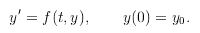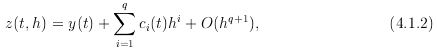.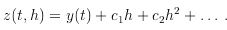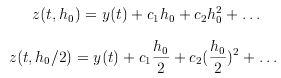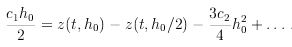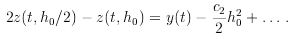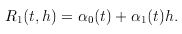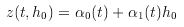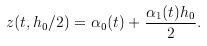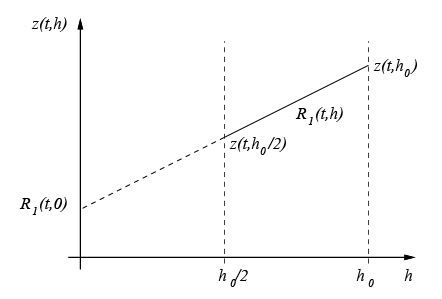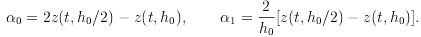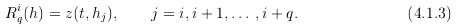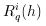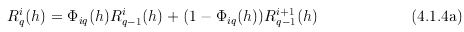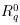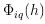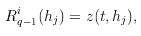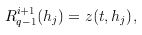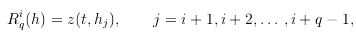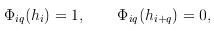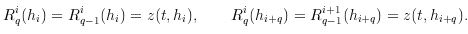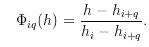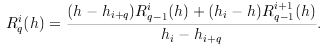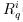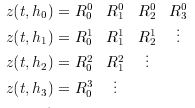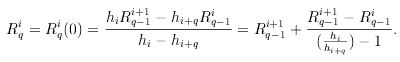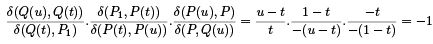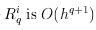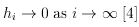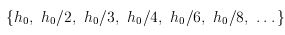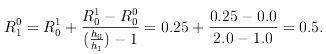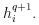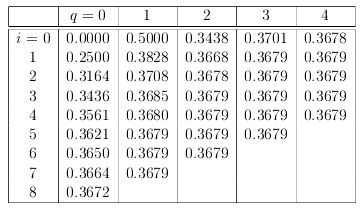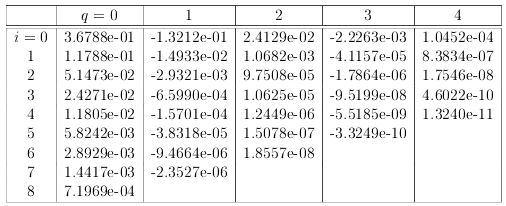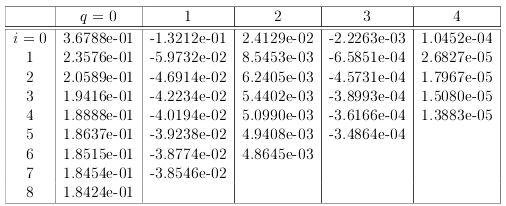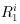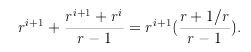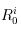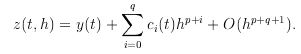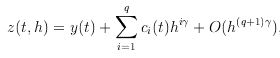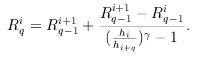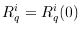Now the order of the approximation is increased by  in each successive column of the extrapolation tableau If the trapezoidal rule is solved exactly at each step then it has an expansion in powers of h       
 Extrapolation with rational functions is also possible      Section II
 The basic idea is to approximate z    t h
by a rational function R    h
and then evaluate R

 Bulirsch and Stoer 
 derived a scheme where Rqi     h
is dened as the rational approximation that interpolates z    t h
at h  hi hi  hiq for h  hi  hi    hiq  The values of Rqi  Rqi

can be obtained from the following recursion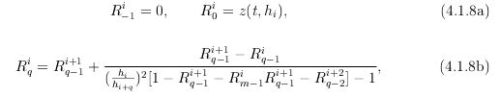when the method has an error expansion in powers of h The computation of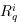ac cording to      
b
is equivalent to interpolating z    t h
by a function of the form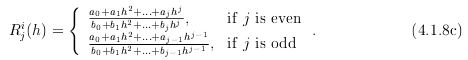There are several enhancements to the basic extrapolation approach Some of these
follow
 A variableorder extrapolation algorithm could choose the order     ie the number of columns in the tableau
adaptively Error estimates can be computed as the dif ference between the rst subdiagonal element and the diagonal or by the dierence between two successive diagonal elements  Extrapolation methods can be written as RungeKutta methods if the step size and order of the extrapolation are xed In this case convergence stability and error bounds follow from the results of Chapter for general onestep methods   Implicit methods can be used in combination with extrapolation to solve sti problems A survey of the state of the art was written by Deuhard   who prefers polynomial extrapolation to rational extrapolation because rational extrapolation lacks translation invariance rational extrapolation can impose restrictions on the base step size and polynomial extrapolation is slightly more ecient

Offer running on EduRev: Apply code STAYHOME200 to get INR 200 off on our premium plan EduRev Infinity!

122 videos|142 docs

,

,

,

,

,

,

,

,

,

,

,

,

,

,

,

,

,

,

,

,

,

,

,

,

;